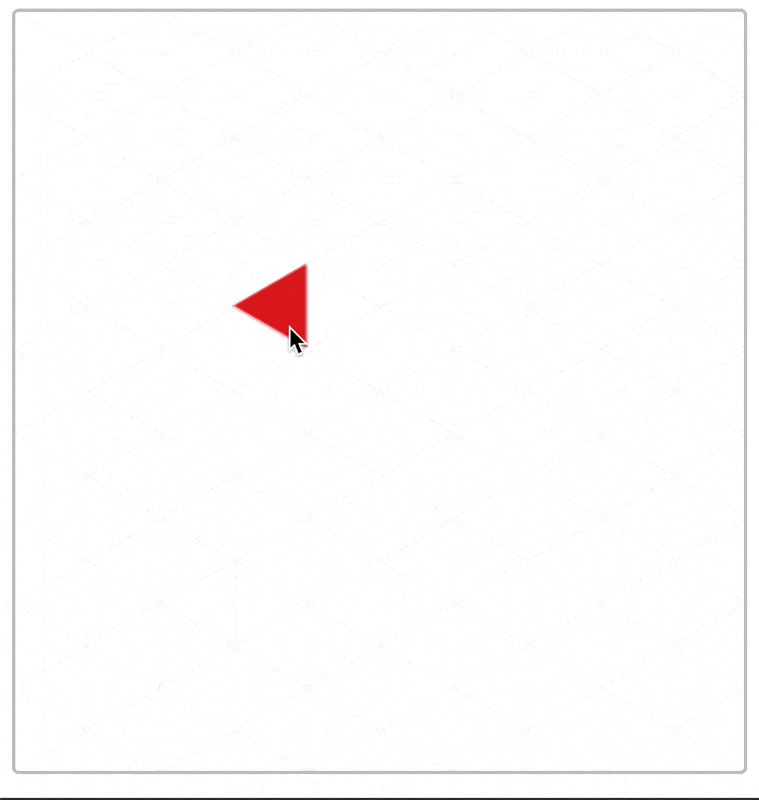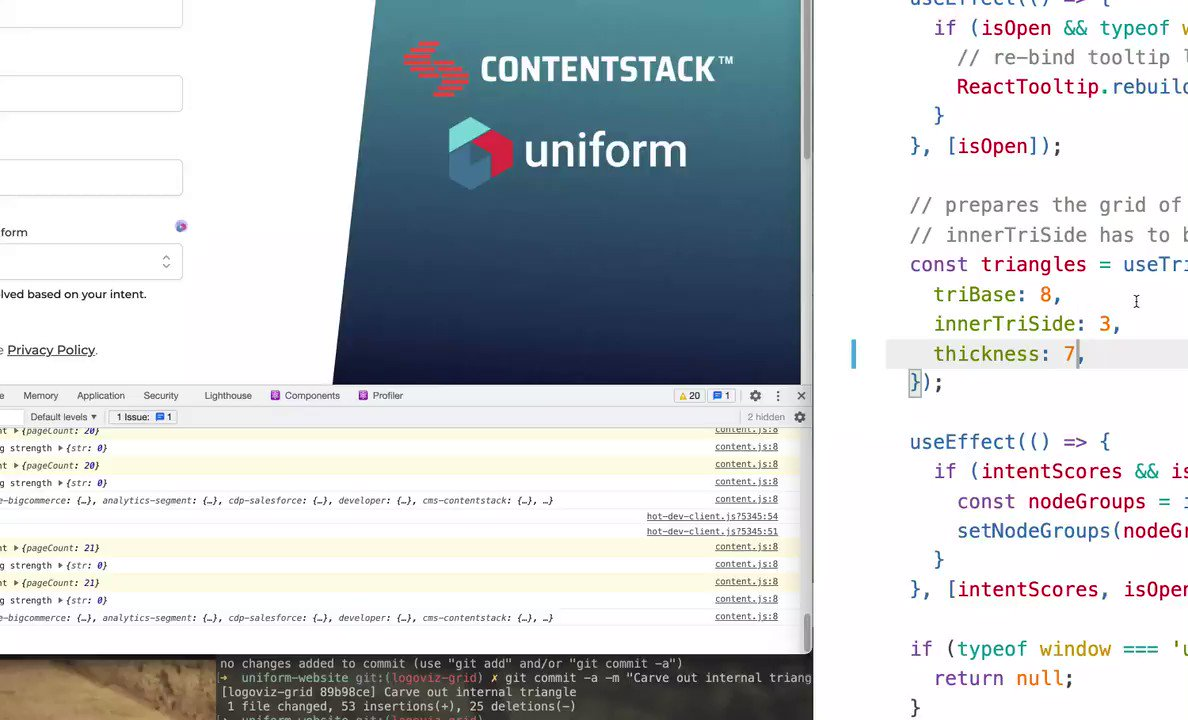swizec.com

## Anything's a sorting problem if you squint hard enough

What's the most algorithmically fun code you've written recently? 🤔

Here's mine: A dataviz using a grid of triangles that fits into the client's logo.Nearly broke my brain. It's good practice.

The dataviz represents your personalization score and attaches to any component that was personalized. Like a form dropdown that guesses your favorite option, or a landing page that chooses copy and pictures you're gonna like.

Each contiguous area of colored triangles represents a score. The number of triangles is proportional to the strength of that score.

The logo designer had a 20 page brief on how the logo is conceptually constructed out of equilateral triangles, can be reconstructed into many shapes, used for visual flourish with conceptual consistency across ... blah

Designers have their fun, engineers have ours.

How the heck do you build this? Are there formulas governing its shape? Where do the triangles come from? Can you cut it up into React components? What about an animated transition ... 🤔

## It starts with triangles

We started with a Codepen hacked in Processing, a visual coding language.This was our playground. A place to explore the rules the grid, how the logo moves, and how far we can push before it starts to look like nothing.

Processing is a readable language, so getting the grid into JavaScript was easy 👉 translate the code.

I made a hook that creates a memoized list of triangles.

```function useTriangles({ triBase }) {  const triHeight = (triBase / 2) * Math.sqrt(3)  const cols = Math.ceil(DATAVIZ_WIDTH / triHeight)  const rows =    Math.ceil(DATAVIZ_HEIGHT / triBase) +    Math.ceil((DATAVIZ_HEIGHT - triBase / 2) / triBase) +    1.css-13aqjzy{display:inline-block;}
// create triangles, memoize  const triangles = useMemo(    () =>      d3        .range(cols)        .map((col) =>          d3.range(rows).map((row) => ({            color: COLORS[(col + row) % 3],            x: col * triHeight + triHeight * ((col + row) % 2),            y: row * (triBase / 2),            dir: Math.round(Math.pow(-1, row + col)),          }))        )        .flat(),    [triBase]  )
return { triangles }}```

`triangles` is a list of triangle objects with:

• `color`, the fill color to use
• `x`, the horizontal coordinate to render at
• `y`, the vertical coordinate
• `dir`, the left/right direction of where the triangle points

Flattening the grid into a list makes rendering easier. A single loop. Calculating `(x, y)` coordinates here makes the rest of your code simpler. Never think about the grid again ✌️

### Render each Triangle

Render each triangle as a `<polygon>` with a React component like this:

`const Triangle = ({ x, y, dir, color, base, height, index, ...rest }) => {  return (    <g transform={`translate(\${x}, \${y})`}>      <polygon        fill={color}        points={`\${0},\${0} \${height * dir},\${base / 2} \${height * dir},\${          -base / 2        }`}      />    </g>  )}`

SVG polygons render from a list of `x,y` points. The math comes straight from your high school textbook.

You get a grid of colored triangles:

My final version adds a `useEffect` to animate each triangle independently. You can learn how that works over at ReactForDataviz.com.

Michelangelo once said that creating a statue is easy – you look at the marble and cut away everything that isn't the statue. D'oh

Carving a shape out of your grid follows the same principle. Except there's a lot of math and it hurts your brain.

Sketches help.I wanted a parametrized shape – thickness, proportions, the size of each triangle. More flexibility when it's time to visualize.

The final result may be the mathiest code I've ever written. There's edge cases where a combination of parameters breaks the shape, but overall works great.

Building this almost broke my brain. Too much math 😅

It's a parametrized shape on top of a grid made of triangles. You have to translate from (row, col) in a 2D array into where you are on triangles then add diagonals

gonna be a cool dataviz tho ✌️Edge cases documented as errors. Good enough 😇

```function useTriangles({ triBase, innerTriSide, thickness }) {  // ...
if (![2, 4, 8, 10].includes(triBase)) {    throw "To avoid jagged edges, triBase has to be in [2, 4, 8, 10]"  }
if (![1, 3, 6, 7, 10].includes(innerTriSide)) {    throw "To avoid jagged edges, the internal blank triangle needs to use sizes in [1, 3, 6, 7, 10]"  }
if (thickness % 2 === 0) {    throw "To avoid jagged edges, thickness needs to be odd"  }
// ...}```

## The final dataviz coloration

You've got the list of colored triangles that make a shape. How do you turn this checkered mess into an organized visualization?

Remember when we turned the grid into a flat list earlier? That's gonna help us now.

You can think of this as a grouping problem – take N triangles for each data you want to represent. Iterate until you run out of triangles.

### Get the groups

D3 scales can calculate group size:

`const proportions = d3  .scaleLinear()  .domain([0, d3.sum(groupScores, (s) => s.str)])  .rangeRound([0, coloredTriangles.length])`

A linear scale that maps `0` to `0` and `sum of all scores` to `number of triangles`. Everything between follows a linear function.

Means you can call `proportions(5)` to see how many triangles that should be. Using that, you go through your data and take N triangles off the grid for each.

```// take proportional triangles from coloredTriangles// merge with scoreconst groups = groupScores.map((score) =>  coloredTriangles.splice(0, proportions(score.str)).map((triangle) => ({    ...triangle,    ...score,  })))
return groups.flat()```

The `score` object has a color property that overrides each triangle's native color. Triangle color was for debugging.

You get a shape full of color groups.

### The .sort gotcha

Now that you're rendering the visually grouped list of triangles, the last step is a lateral thinking exercise. Like those experiments where you get a box of thumbtacks and a candle – attach candle to wall 🤨

Why do the color groups look like vertical slices?

Because triangles come from a grid! The double-loop goes column by column.

You have to sort those triangles before assigning to groups! 🤯

For groups that flow in a weird-shaped circle, you sort triangles by their angle around the center. Looking from the center of each triangle – the centroid.D3 can calculate centroids for us. We'll need 2 – one for each direction of a triangle. Pretend they're at `(0, 0)`.

`;(triCentroidRight = d3.polygonCentroid([  [0, 0],  [triHeight * 1, triBase / 2],  [triHeight * 1, -triBase / 2],])),  (triCentroidLeft = d3.polygonCentroid([    [0, 0],    [triHeight * -1, triBase / 2],    [triHeight * -1, -triBase / 2],  ]))`

Then you attach an `angle` to each triangle and sort.

```const coloredTriangles = triangles.triangles  .filter((t) => t.color !== "transparent")  .map((t) => {    // grab pre-calculated triangle centroid    const centroid = t.dir === 1 ? triCentroidRight : triCentroidLeft
return {      ...t,      // offset centroid by future (x, y) translation      angle: Math.atan2(        centroid + t.y - center.y,        centroid + t.x - center.x      ),    }  })  // sort triangles in a circle around center  .sort((a, b) => {    return b.angle - a.angle  })```

Remove transparent triangles, no need to render those, and use `Math.atan2(y, x)` to get the angle in radians between the `center` of our dataviz and the `centroid` of a triangle.

The `.sort` function sorts our list of triangles by their angle. They're in a circle now.

Group as before and you get the final result – a beautiful dataviz that fits in a logo.Wasn't that fun? It was even more fun to noodle out from scratch.

Point is: Use conventional tools for unconventional means.

Cheers,
~Swizec

PS: to solve the candle problem, you have to realize that the box is an item separate from thumbtacks. You can use it as a shelf for the candle.

Published on September 7th, 2021 in Technical, Dataviz, Algorithms

## Learned something new? Want to become an expert?

Here's how it works 👇

Leave your email and I'll send you thoughtfully written emails every week about React, JavaScript, and your career. Lessons learned over 20 years in the industry working with companies ranging from tiny startups to Fortune5 behemoths.

And get thoughtful letters 💌 on mindsets, tactics, and technical skills for your career. Real lessons from building production software. No bullshit.

"Man, love your simple writing! Yours is the only newsletter I open and only blog that I give a fuck to read & scroll till the end. And wow always take away lessons with me. Inspiring! And very relatable. 👌"

Join over 10,000 engineers just like you already improving their careers with my letters, workshops, courses, and talks. ✌️

Have a burning question that you think I can answer? I don't have all of the answers, but I have some! Hit me up on twitter or book a 30min ama for in-depth help.

Ready to Stop copy pasting D3 examples and create data visualizations of your own?  Learn how to build scalable dataviz components your whole team can understand with React for Data Visualization

Curious about Serverless and the modern backend? Check out Serverless Handbook, modern backend for the frontend engineer.

Ready to learn how it all fits together and build a modern webapp from scratch? Learn how to launch a webapp and make your first 💰 on the side with ServerlessReact.Dev

Want to brush up on your modern JavaScript syntax? Check out my interactive cheatsheet: es6cheatsheet.com

By the way, just in case no one has told you it yet today: I love and appreciate you for who you are ❤️

Created bySwizecwith ❤️